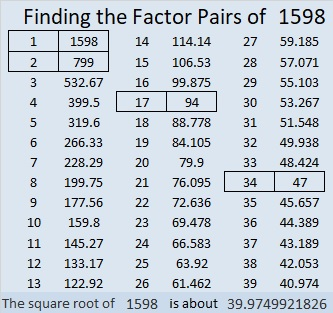# 1598 See the Logic in This Level 4 Puzzle

Contents

### Today’s Puzzle:

Put the numbers from 1 to 10 in both the first column and the top row to turn this level 4 puzzle into a multiplication table. The logic in the ten clues is fairly straight-forward. Go ahead give it a try!### Factors of 1598:

• 1598 is a composite number.
• Prime factorization: 1598 = 2 × 17 × 47.
• 1598 has no exponents greater than 1 in its prime factorization, so √1598 cannot be simplified.
• The exponents in the prime factorization are 1, 1, and 1. Adding one to each exponent and multiplying we get (1 + 1)(1 + 1)(1 + 1) = 2 × 2 × 2 = 8. Therefore 1598 has exactly 8 factors.
• The factors of 1598 are outlined with their factor pair partners in the graphic below.### More about the Number 1598:

1598 is the hypotenuse of a Pythagorean triple:
752-1410-1598, which is (8-15-17) times 94.

This site uses Akismet to reduce spam. Learn how your comment data is processed.